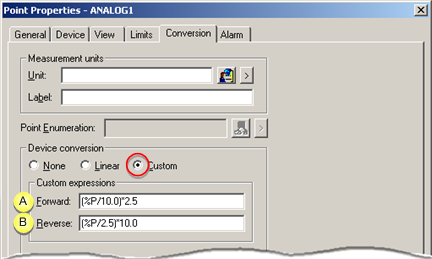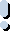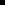##### Option 3.2.3. Custom Conversion

Custom conversion specifies specific forward and backward conversion expressions.

 Custom conversion configuration. Valid operations. Expression processor interpretation.

Custom conversion configuration

1. Check Custom in the Device conversion box.

2. Enter values as follows.A Forward Converts the raw data to engineering units. Use the placeholder %P to indicate the point value in the expression. %P is the raw value of the point. B Reverse Converts set point values from engineering units to raw data. Use the placeholder %P to indicate the point value in the expression. %P is the engineering units value of the point.

Valid Operations

Forward and Reverse operations use the functions that are available in the Expression Editor .

Example

A conversion calls for the raw data to be divided by 10.0 then multiplied by 2.5.

The Forward expressions is (%P/10.0)*2.5

The Reverse expression is (%P/2.5)*10.0

Expression processor interpretationImportant: When creating a custom conversion:The numeric operands you use in the Forward expression are assumed to be integers unless you include decimal points in them. Once the expression processor sees an operand with a decimal point, it will process the rest of the expression using floating-point arithmetic.A conversion that inverts the sign, (e.g. a FWD Conversion = %P(-1) and REV Conversion = %P(-1)) will not work correctly if you configure a set point, range or alarm limits

Example

A conversion that calls for the raw data to be divided by 2, then multiplied by 10.

The raw value for the point is 3.

You could enter any one of the following expressions. However, the Expression Processor interprets each expression differently, as follows:

 Expression Result Because integer arithmetic was used throughout, the result is an integer: (%P/2)*10 (3/2)*10 = (1)*10 = 10 Because integer arithmetic was used in the first operation of these two expressions, the operation (3/2) gives the result 1 instead of 1.5: (%P/2)*10.0 (3/2)*10.0 = (1)*10.0 = 10.0 Because (3/2.0) is processed using floating point arithmetic, the rest of the expression will be processed using floating point arithmetic, even thought 10 is entered as an integer: (%P/2.0)*10 (3/2.0)*10 = (1.5)*10 = 15.0 Because (3/2.0) is processed using floating-point arithmetic, the rest of the expression will be processed using floating-point arithmetic: (%P/2.0)*10.0 (3/2.0)*10.0 = (1.5)*10.0 = 15.0
 Step 3.2. Configure the conversion type for a device point.